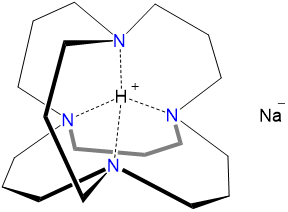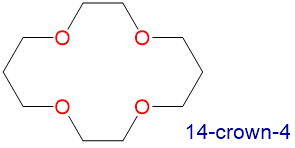# 8.3.1: Alkali Metals' Chemical Properties

## The chemistry of the alkali metals reflects their tendency to form +1 cations.

The alkali metals tend to form +1 cations. Cation formation is favored by the relatively low ionization energies of the free metal (which makes it easier to form the cation) and the high solvation energy of their cations (which indicates that the cation is thermodynamically stabilized in solution). The variations in ionization energy and solvation energies down the alkali metal group help explain why Li is more reducing than the other alkali metals.1 The relevant ionization energies and hydration enthalpies are given in Table $$\sf{\PageIndex{1}}$$. As can be seen from the data in Table $$\sf{\PageIndex{1}}$$, the ionization energy of the metal decreases by ~20% on going from Li to Cs while the solvation energy of the cation decreases by ~75% over the same range (with ~2/3 of that decrease taking place on going from Li+ to Na+).

Table $$\sf{\PageIndex{1}}$$. Selected thermodynamic properties of alkali metals and their cations.2,3

 Alkali Metal Ionization energy (kJ/mol) Solvation enthalpy of the +1 Cation (kJ/mol) Lithium, Li 513 -1091 Sodium, Na 496 -515 Potassium, K 419 -405 Rubidium, Rb 403 -321 Cesium, Cs 376 -296 Francium. Fr 400 -263

One result of this greater stability of Li+ in solution relative to that of other alkali metal cations is that Li tends to be the most reducing of the alkali metals, as illustrated by the reduction potentials of the metals given in Figure $$\sf{\PageIndex{1}}$$. Nevertheless, as can be seen in Figure $$\sf{\PageIndex{1}}$$, the reduction potentials of the other alkali metals do not monotonically increase on going from Na to Cs. Instead, Na is the least reducing metal while the reduction potentials of K, Rb, and Cs are approximately equal.Figure $$\sf{\PageIndex{1}}$$. Standard reduction potentials in volts for reduction of selected aqueous cations in acid solution.

## Because of their tendency to form cations, alkali metals are effective reducing agents.

Because of their tendency to form cations, alkali metals are highly reducing. All react vigorously with water to give hydroxides.

$\sf{2~M(s)~~+~~2~H_2O(l)~\rightarrow~2~M^+~~+~~2~OH^-~~+~~H_2(g)~~~~~~(M~=~= Li,~Na,~K,~Rb,~Cs)}$

The reactions are all exothermic and often lead to combustion of the evolved H2 gas. As a result, while Li usually just fizzes as it reacts, Na and K usually result in the formation of a colored flame while the reactions of Rb and Cs result in an explosion.

More synthetically useful is the reduction of alkyl halides by Li metal to give organolithium reagents.

$\sf{R-Cl~~+~~2~Li(s)~~\overset{THF}{\longrightarrow}~~RLi~~+~~LiCl}$

Under conditions where the metal cation is stabilized in the absence of an oxidizing agent it is even possible to form salts of free or solvated electrons. Small amounts of sodium may be dissolved in dry donor solvents (ammonia, tertiary amines, hexamethylphosphoramide) to give paramagentic blue solutions containing solvated electrons.

$\sf{Na(s)~~\overset{NH_3}{\longrightarrow}~~Na(NH_3)_6^+~~+~~e^-(solv.)~~~~(at~low~Na^+~concentrations)}$

At higher concentrations of sodium bronze solutions containing sodide anions (e.g. M-) are formed.

$\sf{2~Na(s)~~\overset{NH_3}{\longrightarrow}~~Na(NH_3)_6^+~~+~~Na^-(solv.)~~~~(at~high~Na^+~concentrations)}$

As either electrides or sodides the solutions are excellent reducing agents. In some cases, they can even be used to reduce metals to negative oxidation states.

$\sf{Na(NH_3)_6^+~~+~~e^-(solv.)~~+~~Au(s)~~\longrightarrow~~Na(NH_3)_6^+~~+~~Au^-(solv.)}$

In the presence of a catalyst the solvated electron decomposes by reducing the ammonia.

$\sf{2~e^-(solv.)~~+~~2~NH_3~~\overset{catalyst}{\longrightarrow}~~2~NH_2^-~~+~~H_2}$

Synthetically, sodium-ammonia solutions are useful for preparing acetylides and reduced organometallic complexes.

$\sf{R-C≡C-H~~+~~Na^+(solv.)~~+~~e^-(solv.)~~\longrightarrow~~R-C≡C:^-Na^+~~+~~{\textstyle \frac{1}{2}}~H_2}$

$\sf{Fe(CO)_5~~+~~2~Na^+(solv.)~~+~~2~e^-(solv.)~~\longrightarrow~~(Na^+)_2[Fe(CO)_5^{2-}]~~+~~{\textstyle \frac{1}{2}}~H_2}$

Solid electride salts - i.e. in which the anion is a free electron - may even be prepared by adding a ligand that encapsulates and stabilizes the metal cation and then allowing the solvent to evaporate away from the solvated electron.

$\sf{Na(NH_3)_6^+~~+~~e^-(solv.)~~+~~encapsulating~ligand~~\underset{-6~NH_3}{\longrightarrow}~~Na(encapsulating~ligand)^+e^-(s)}$

Most of the resulting solid electride salts are only stable at low temperatures. The electron density of the free electron "counterions" in crystalline electrides is thought to be localized in cavities within the structure.

The anion of sodium, sodide, Na-, can be formed be reducing the alkali metal under suitable conditions. Among the more remarkable alklai metal anions salts is "inverse sodium hydride."  Inverse sodium hydride is formally a H+ salt of Na- in which the H+ ion is encapsulated in an adamanzane cage (Figure $$\sf{\PageIndex{2}}$$. This encapsulation slows the thermodynamically favorable reduction of H+ by the sodide anion, stabilizing the inverse sodium hydride long enough to permit its isolation.

Scheme $$\sf{\PageIndex{I}}$$. "Inverse sodium hydride" (i.e. H+Na-).## The chemistry of the alkali metals depends on the size of the alkali metal cations.

The stability of alkali metal compounds depends on the stabilization of their +1 cations. This stability in turn depends on the size of the cations. Alkali metal cation size helps determine the structure and in some cases the identity of the compound that a given alkali metal forms with a given nonmental. An example of this involves the structures formed by the alkali metal halides. The halides of smaller alkali metal cations - Li+, Na+, K+, and Rb+ - crystallize in the rock salt structure with six halide ions about each ion (Figure $$\sf{\PageIndex{2A}}$$). In contrast, the chlorides, bromides, and iodides of the larger Cs+ ion crystallize in the CsCl structure, in which there are six halides about the larger Cs+ ion (Figure $$\sf{\PageIndex{2B}}$$).Figure $$\sf{\PageIndex{2}}$$. (A) Rock salt structure formed by LiX, NaX, KX, and RbX (X = F, Cl, Br, and I) showing octahedral coordination of six halides about each metal ion. (B) CsCl structure formed by CsCl, CsBr, and CsI showing square coordination of eight halides about each Cs atom. The rock salt structure is by Solid State - Own work, Public Domain, commons.wikimedia.org/w/inde...?curid=3837309 ;the CsCl structure image by Solid State - Own work, Public Domain, commons.wikimedia.org/w/inde...?curid=3837330

Size effects are also believed to be responsible for alkali metal's unusual tendency to form peroxides and superoxides when burned in an excess of oxygen. While most metals form oxides hen burned in an excess of oxygen, Li and Na also form 2:1 salts of the larger peroxide ion (O22-) and K, Rb, and Cs 1:1 salts of the similarly large superoxide ion (O2-) (Figure $$\sf{\PageIndex{3}}$$).Figure $$\sf{\PageIndex{3}}$$. Products formed when metals are heated in excess of dry O2 showing the increasing preference of larger alkali metals to form peroxides and superoxides.

Perhaps the most striking examples of size effects in alkali metal chemistry involve alkali metal complexes with macrocyclic ligands, particularly crown ethers and cryptands like those shown in Figure $$\sf{\PageIndex{4}}$$.Figure $$\sf{\PageIndex{4}}$$. Structures of typical crown ether and cryptand complexes. (A) Cyclic ethers like 18-crown-6 can chelate metal ions through their oxygen lone pairs. They are called crown ethers3 because of they take on a crownlike puckered appearance when bound to the metal, as may be seen from (B) the coordination of 18-crown-6 and sulfate to Cs+ in the structure of Cs[H2PO4]0.5[HSO4]0.5·3H2O in which the crown ether "crowns" the Cs+ ion by binding to one side of it. This leaves the Cs+ free to bind additional ligands like SO42-. (C) In contrast to crown ethers, cryptands like 2.2.2-crypt4 consist fo two amines connected by three polyether chains. This allows them to completely encapsulate or "entomb" metals, (D) as may be seen by the coordination of 2.2.2.cryptan to K+ in (2.2.2-Cryptand)potassium tetra­carbonyl­cobaltate(−1). Key: grey = C; white = H; red = O; yellow = S; blue = Cs; and violet = K+. The structures in B and C are drawn from the cif files reported in references 8 and 9, respectively.

Crown ethers and cryptands prefer to bind alkali metal cations with sizes that match that of their binding cavity. For instance, as shown in Table $$\sf{\PageIndex{2}}$$, 14-crown-4 preferentially binds to Li+, 15-crown-5 preferentially binds to Na+, 18-crown-6 preferentially binds to K+, and 21-crown-7 preferentially binds to Cs+.

Table $$\sf{\PageIndex{2}}$$. Crown ethers preferentially bind cations with sizes that match the cavity size of their crown-shaped conformers. Cavity and cation diameters are taken from reference 10.

 Crown ether ...with a cavity diameter of (in $$\mathring{\mathrm{A}}$$ ) ...preferentially binds ...which has a cation diameter of (in $$\mathring{\mathrm{A}}$$ )1.2-1.5 Li+ 1.361.7-2.2 Na+ 1.942.6-3.2 K+ 2.663.4-4.3 Cs+ 3.34

Exercise $$\PageIndex{1}$$

Cryptand ligands also preferentially bind alkali metal ions with sizes that match the size of their binding cavities. The structure and approximate cavity sizes of several cryptands are given in Scheme$$\sf{\PageIndex{II}}$$. Use the information in Scheme$$\sf{\PageIndex{II}}$$ and Table $$\sf{\PageIndex{2}}$$ along with a cation diameter of 2.98 Å for Rb+ to predict which alkali metal ion each cryptand will preferentially bind.

Scheme $$\sf{\PageIndex{II}}$$. Structures and approximate binding cavity diameters for several cryptands.The cryptands might be expected to selectively bind the largest ion which fits within the cavity. These predictions are summarized in Table $$\sf{\PageIndex{3}}$$.

Table $$\sf{\PageIndex{3}}$$. Predicted selectivity of crown ethers for alkali metal cations based on the hypothesis that they will selectively bind the largest ion which fits their bindng cavity.

 Cryptand ...with a cavity diameter of (Å) ...will be selective for ...with a radius of (in Å) 2.1.1-cryptand 1.60 Li+ 1.36 2.1.1-cryptand 2.20 Na+ 1.96 2.1.1-cryptand 2.80 K+ 2.66 2.1.1-cryptand 3.60 Cs+ 3.34

These predicted selectivities match the experimental observations with one exception. It turns out that 2.1.1-crypt preferentially binds the smaller Rb+ ion over Cs+, although the difference in binding constant is a small one (for more details see reference 12). One reason for this is the flexibility of the cryptand ring system, which allows the cryptand oxygen and nitrogen Lewis base sites to adjust position to accommodate the needs of each alkali metal. In fact, because of this flexibility all four cryptands can bind all the alkali metals, even those that are nominally too large to fit the cryptand's optimal cavity diameter.

The ability of flexibile macrocycles like cryptands and crown ethers to change conformation when binding a Lewis acid means that the cavity size estimates given in Scheme$$\sf{\PageIndex{II}}$$ are of limited utility predicting the relative binding strengths.

## References

1. Since enthalpies of solution involve the dissolution of a solid and ionization energies the ionization of gas phase atoms a rigorous analysis should also account for the energy associated with sublimation.

2. Ionization energies are taken from Emsley, J. The elements 2nd ed. Oxford University Press, 1991.

3. Hydration enthalpies are converted to kJ/mol from the values given in Burgess, J. Metal ions in solution Halsted Press: Chichester
New York, 1978; pp. 182-183.

4. Crown ethers are named x-crown-y after the total number of atoms in the ring, x, and the number of oxygen ligand sites, y. Thus the ring of 18-crown six contains a total of 18 atoms, 6 O and 12 C.

5. Cryptands are named x.y.z.-crypt after the number of oxygen binding sites in each of the chains connecting the two amine groups. Thus 2.2.2-crypt consists of two amines connected by three diether chains.

6. Bard, A. J.; Parsons, R.; Jordan, J.; International Union of Pure and Applied Chemistry., Standard potentials in aqueous solution. 1st ed.; M. Dekker: New York, 1985.

7. Redko, M. Y.; Vlassa, M.; Jackson, J. E.; Misiolek, A. W.; Huang, R. H.; Dye, J. L., “Inverse Sodium Hydride”:  A Crystalline Salt that Contains H+ and Na. Journal of the American Chemical Society 2002, 124 (21), 5928-5929.

8. Braga, D.; Modena, E.; Polito, M.; Rubini, K.; Grepioni, F., Crystal forms of highly “dynamic” 18-crown complexes with M[HSO4] and M[H2PO4] (M+ = NH4+, Rb+, Cs+): thermal behaviour and solid-state preparation. New Journal of Chemistry 2008, 32 (10), 1718-1724.

9. Brennessel, W. W.; Ellis, J. E., (2.2.2-Cryptand)potassium tetra-carbonyl-cobaltate(-I). Acta Crystallogr Sect E Struct Rep Online 2014, 70 (Pt 5), m180-m180.

10. Pederson, C.J. "Synthetic Multidentate Macrocyclic Compounds" in Izatt, R. M.; Christensen, J. J., (eds.) Synthetic multidentate macrocyclic compounds. Academic Press: New York, 1978; pp. 24-25.

11. Wagner, M. J.; Dye, J. L., Alkalides, Electrides, and Expanded Metals. Annual Review of Materials Science 1993, 23 (1), 223-253.

12. Dye, J. L., Electrides, Negatively Charged Metal Ions, and Related Phenomena. In Progress in Inorganic Chemistry, Lippard, S. J., Ed. Wiley: 1984; pp 327-441.